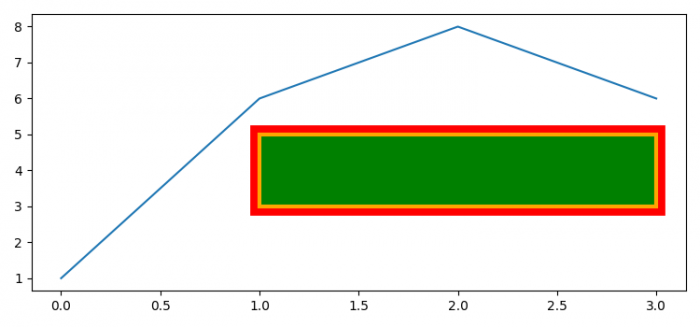# How to set a Matplotlib rectangle edge to outside of specified width?

MatplotlibPythonData Visualization

#### Python Data Science basics with Numpy, Pandas and Matplotlib

Most Popular

63 Lectures 6 hours

#### Data Visualization using MatPlotLib & Seaborn

11 Lectures 4 hours

#### MatPlotLib with Python

9 Lectures 2.5 hours

To set a Matplotlib rectangle edge to outside of specified width, we can take the following steps −

• Set the figure size and adjust the padding between and around the subplots.
• Create a new figure or activate an existing figure.
• Add an ax to the figure as part of a subplot arrangement.
• Initialize a variable line_width to set the rectangle outside of specified width. Use the variables xy, w and h for rectangle's center, width and height.
• Get a rectangle instance, with xy anchor points and its height and width.
• Get the offset transformbox instance.
• Add an artist patch, r (Step 5).
• Get the container for an OffsetBox instance.
• Add an annotation box, ab, to the container box.
• To display the figure, use show() method.

## Example

import matplotlib.pyplot as plt
from matplotlib.patches import Rectangle
from matplotlib.offsetbox import AnnotationBbox, AuxTransformBox

plt.rcParams["figure.figsize"] = [7.50, 3.50]
plt.rcParams["figure.autolayout"] = True

fig = plt.figure()

ax.plot([1, 6, 8, 6])

line_width = 7
xy, w, h = (1, 3), 2, 2
r = Rectangle(xy, w, h, fc='green', ec='orange', lw=3)

offsetbox = AuxTransformBox(ax.transData)

ab = AnnotationBbox(offsetbox, (xy + w /, xy + w /),
plt.show()